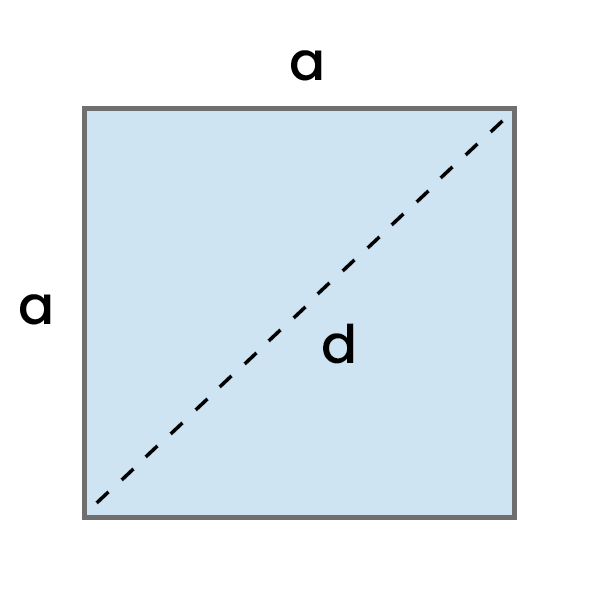# Perimeter of a Square Calculator

Calculate the perimeter of a square based on the entered side length or diagonal length in the chosen units.Result:

## How to use this calculator?

1. Enter the length of side "a" or the length of the diagonal "d" in your chosen unit.
2. See the result and optionally change its unit.

You can also check the calculation process below the result.

## How to calculate the Perimeter of a Square?

To calculate the perimeter of a square, multiply the length of one side by four.

P = a * 4
If you don't know the length of "a", you can obtain it based on the diagonal. Just divide the length of the diagonal by the square root of two.
a = d / √2

## Examples

Example 1: Calculate the perimeter of a square with a side of 5 cm.

P = a * 4

P = 5 cm * 4

P = 20 cm

Example 2: Calculate the perimeter of a square with a diameter of 8 in.

a = d / √2

a = 8 in / √2

a = 5.66 in

P = a * 4

P = 5.66 in * 4

P = 22.64 in